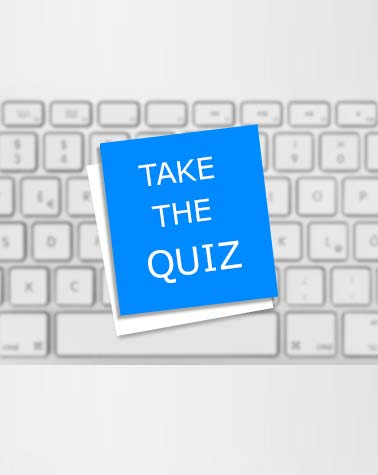# Basic Excel Quiz For Learners!

8 QuestionsSettingsSMAN1 Bunguran Timur Laut

Related Topics
• 1.
What is an cell?
• A.

In a spreadsheet, the intersection of a row and column.

• B.

An elementary unit of storage for data (bit) or power (battery).

• C.

A geographic area in a cellular phone system. See cellphone.

• 2.
A cell pointer is a rectangular highlight that indicates the active cell in a spreadsheet program.
• A.

True

• B.

False

• 3.
• 4.
A colunm one of two or more vertical sections of typed lines lying side by side on a page and separated by a rule or a blank space.
• A.

True

• B.

False

• 5.
What is the correct definition of formula?
• A.

An arithmetic expression that solves a problem. For example, (fahrenheit-32)*5/9 is the formula for converting Fahrenheit to Celsius

• B.

In spreadsheets, an algorithm that identifies how the data in a specific number of cells is to be calculated.

• C.

A means or method of entering into or achieving something desirable

• 6.
Define the words fnction,range,row,and workbook.*Key the definitions must relate to microsoft exel.
• 7.
Software that simulates a paper spreadsheet (worksheet), in which columns of numbers are summed for budgets and plans. It appears on screen as a matrix of rows and columns, the intersections of which are called "cells." The cells are filled with (1) labels, (2) numeric values or (3) formulas.
• A.

True

• B.

False

• 8.
Select the cells you want to merge. There is a tool on the formatting toolbar that is called merge and center that you can then click. It looks like an a with arrows pointing out of it. You can also go to the format menu, pick cells, then alignment and then tick merge cells.
• A.

True

• B.

False